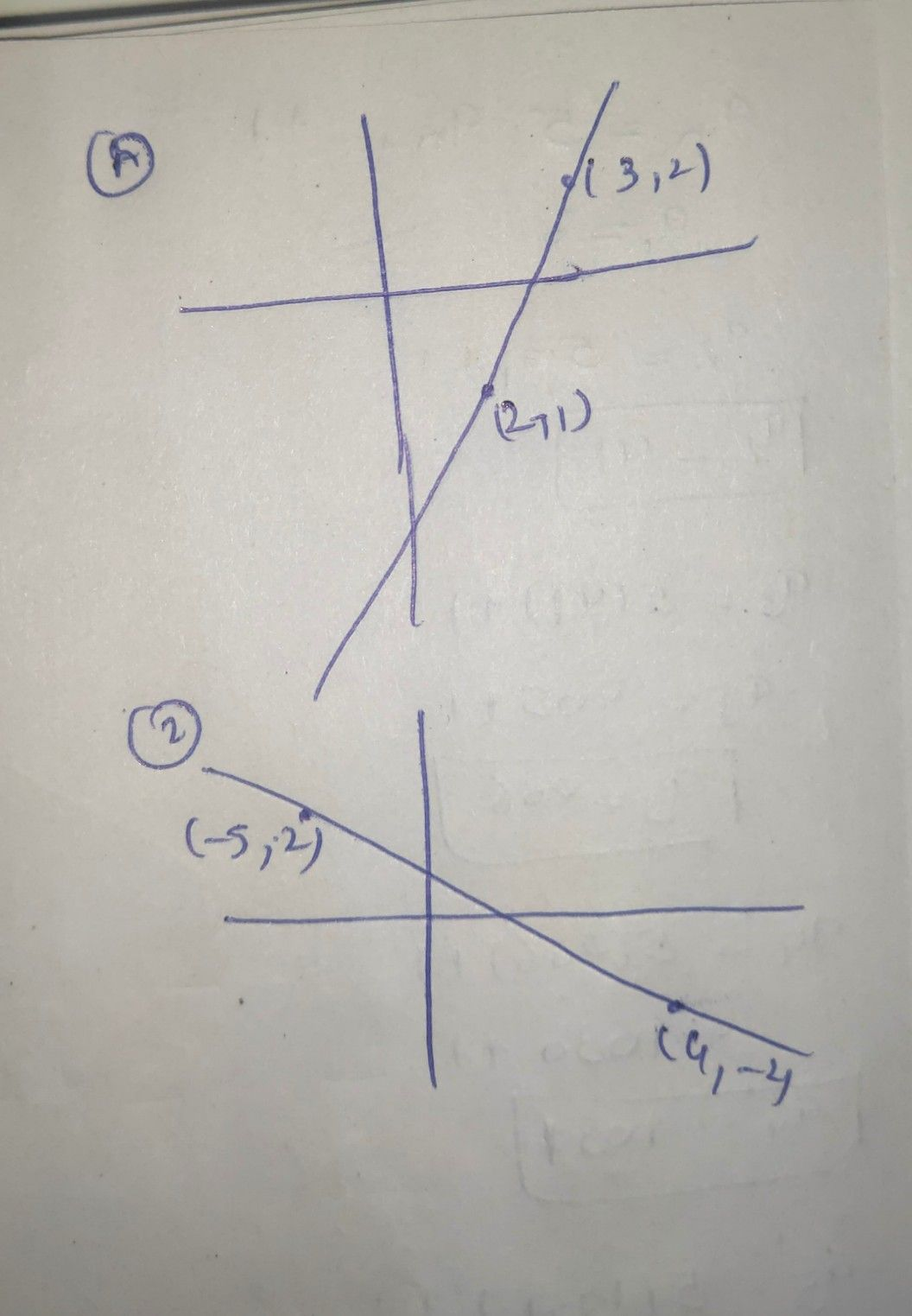Symbol
ProblemGraph the linear equations given the following $details:$ A. Using two points. $1.$ $\left(2,-1\right)$ and $\left(3,2\right)$ $3.$ $\left(4,3\right)$ and $\left(0,$ $5\right)$ $2$ $\left(4,-2\right)$ and $\left(-5,$ $2\right)$ $4$ $\left(1,6\right)$ and $\left(-2,$ $-5\right)$ Find the slope of each line . Describe the graph.
7th-9th grade
Other
Search count: 105
SolutionQanda teacher - Askhina